Learn data science and machine learning by building real-world projects on Jovian

Course Project on EDA with Python

Analysis on Video Game Dataset

## Exploratory analysis on Video Games Sales data

Name : ABUBAKKAR SIDDIQUE

Qualification : Bachelor of Engineering (BE)

Stream : Computer Science and Engineer (CSE)

University : Visvesvaraya Institute of Technology

Location : Bangalore, KA, INDIA

This Project is to perform the analysis on the Video Games Sales dataset. Here we use various libraries of Python for visualization of Data. The Dataset which is Used in Project is from Data World (👈 Click to Download)

The Libraries I used in Project are:

To install all required libraries, run the following Command:

`pip install matplotlib seaborn numpy pandas plotly jovian --upgrade`

The Data visualization is the graphic representation of data. It involves producing images that communicate relationships among the represented data to viewers. Visualizing data is an essential part of data analysis and machine learning. In this tutorial, we'll use Python libraries Matplotlib and Seaborn to learn and apply some popular data visualization techniques.

Their Following Tasks are Implemented in the Project:

### Let's Get Dive into the Project !!

In :
``project_name = "analysis-on-videogames-sales-data"``
In :
``!pip install jovian --upgrade -q``
In :
``````import jovian
import matplotlib.pyplot as plt
import seaborn as sns
import plotly.graph_objs as go
import numpy as np
from plotly.offline import init_notebook_mode,iplot
import pandas as pd
%matplotlib inline
``````

## Data Preparation and Cleaning

Here various modes of displaying dataset which is in CSV format. First step is to load the data using pandas read_csv function. the data is stored in mutidimensional table called as dataframe.

In :
``````data = 'VideoGameSales.csv' #locate the CSV dataset in variable data

videogame_df = pd.read_csv(data) #read the data using pandas and store it in videogame_df variable
videogame_df #display the data (completely )``````
Out:

This Cell is to Explain the details of all Columns :

• Ranking -- Game ranking based on the total sales (in millions)
• Name -- Name of the Game
• Platform -- Game Platforms like (PS4, PC, GB etc)
• Year -- Year of game release
• Genre -- Simply the game genre (sports, racing ... )
• publisher -- name of the publisher
• NA_Sales -- Sales in north america (in millions)
• EU_sales -- Sales in Europe (in millions)
• JAP_sales -- Sales in Japan (in millions)
• IND_Sales -- Sales in India (in millions)
• Global_Sales -- Total sales world wide (in millions)
In :
``videogame_df.describe()``
Out:

From above Dataframe, we conclude that :

• 500 games are ranked based on their sales
• Games released between 1980 to 2020
• Mean/Average sales in all regions are very low compare to the Max ...
In :
``videogame_df.shape #To display the shape of the data (rows, columns)``
Out:
``(500, 11)``
In :
``videogame_df.sort_values(by = ['Name']).head(30) #Display top 30 rows and Sort by 'Name' column``
Out:
In :
``videogame_df.head(10) #To display top 10 rows from the dataset``
Out:
In :
``videogame_df.tail(10) #Display 10 rows from bottom of dataframe``
Out:
In :
``videogame_df[50:60] #Display the rows in range from 51 to 60``
Out:

### To Print all Columns names

In :
``````#Method 1 to print all column names
for col in videogame_df.columns:
print(col)

#Method 2 to print all col names
list(videogame_df.columns) ``````
```Rank Name Platform Year Genre Publisher NA_Sales EUR_Sales JAP_Sales IND_Sales Global_Sales ```
Out:
``````['Rank',
'Name',
'Platform',
'Year',
'Genre',
'Publisher',
'NA_Sales',
'EUR_Sales',
'JAP_Sales',
'IND_Sales',
'Global_Sales']``````
In :
``````x = videogame_df['Name'].unique() #using numpy.ndarray to find all Names but only UNIQUE.
y = videogame_df['Genre'].unique()
z = videogame_df['Publisher'].unique()``````
In :
``````print('Total Games by `Name` count(unique) :',len(x))
print('Total Games by `Genre` count(unique) :',len(y))
print('Total Games by `Publisher` count(unique) :',len(z))

``````
```Total Games by `Name` count(unique) : 431 Total Games by `Genre` count(unique) : 12 Total Games by `Publisher` count(unique) : 34 ```

## Exploratory Analysis and Visualization

#### Few randomly created analysis on Video Game sales Dataset

Now, to use Matplotlib, Seaborn library to visualize the Dataset.

In :
``````vg_plot = videogame_df[0:25]
vg_plot
``````
Out:
In :
``````x = vg_plot['Rank']
y = vg_plot['Year']
plt.figure(figsize=(25,8), dpi= 80)
plt.plot(x,y, label = 'Year', color = 'green')
plt.xlabel('Rank')
plt.ylabel('Year')
plt.title('Global Sales by Rank For 25 Rows')
plt.legend()
plt.show()
``````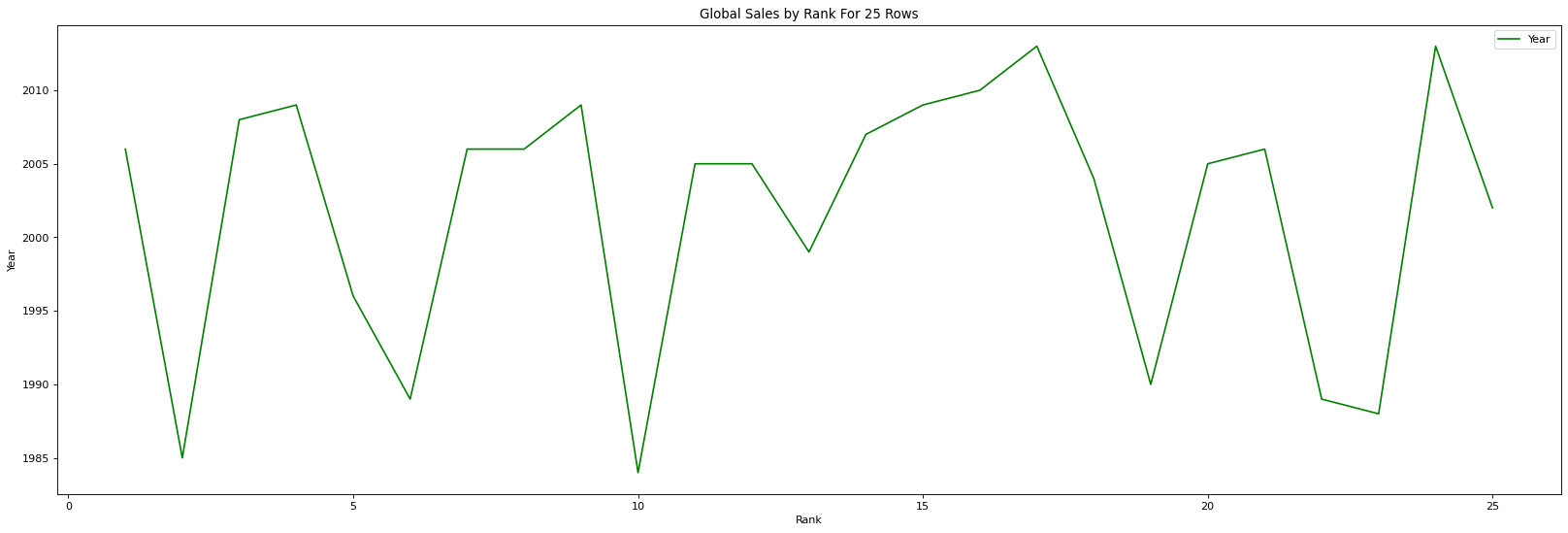### Seaborn's kdeplot

Now we can also get a smooth estimate of the distribution using a kernel density estimation, which Seaborn does with sns.kdeplot

In :
``````# Draw Plot
plt.figure(figsize=(25,8), dpi= 80)
sns.kdeplot(videogame_df.Global_Sales, shade=True, label = 'Global Sales', color="r", alpha=.7)

# Decoration
plt.title('Overall Global Sales Distribution', fontsize=16)
plt.legend()
plt.show()
``````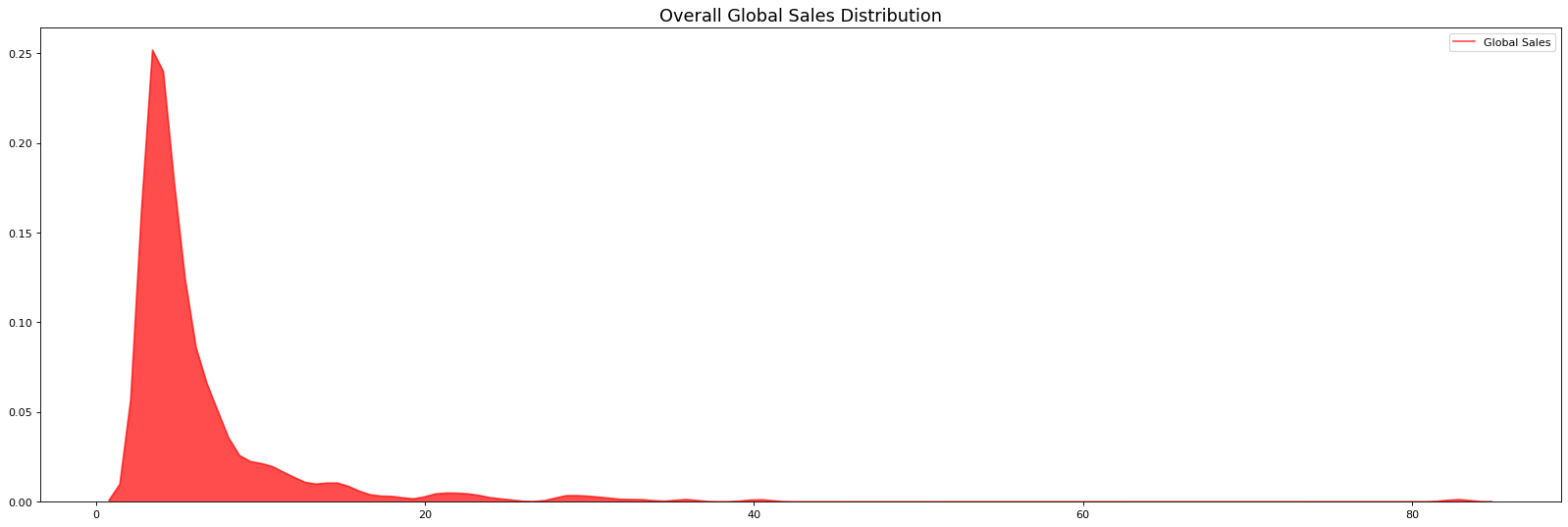In :
``````total = vg_plot['Global_Sales']
NA = vg_plot['NA_Sales']
EUR = vg_plot['EUR_Sales']
JAP = vg_plot['JAP_Sales']
IND = vg_plot['IND_Sales']``````
In :
``````plt.figure(figsize=(25,8), dpi= 80)
plt.grid(True)
plt.title('Comparision With all Countries with Global Sales')

plt.plot(total, label = 'Global')
plt.plot(NA, label = 'AMERICA')
plt.plot(EUR, label = 'EUROPE')
plt.plot(JAP, label = 'JAPAN')
plt.plot(IND, label = 'INDIA')
plt.legend(bbox_to_anchor =(1.0, 1.025), ncol = 2)``````
Out:
``<matplotlib.legend.Legend at 0x23389a92cd0>``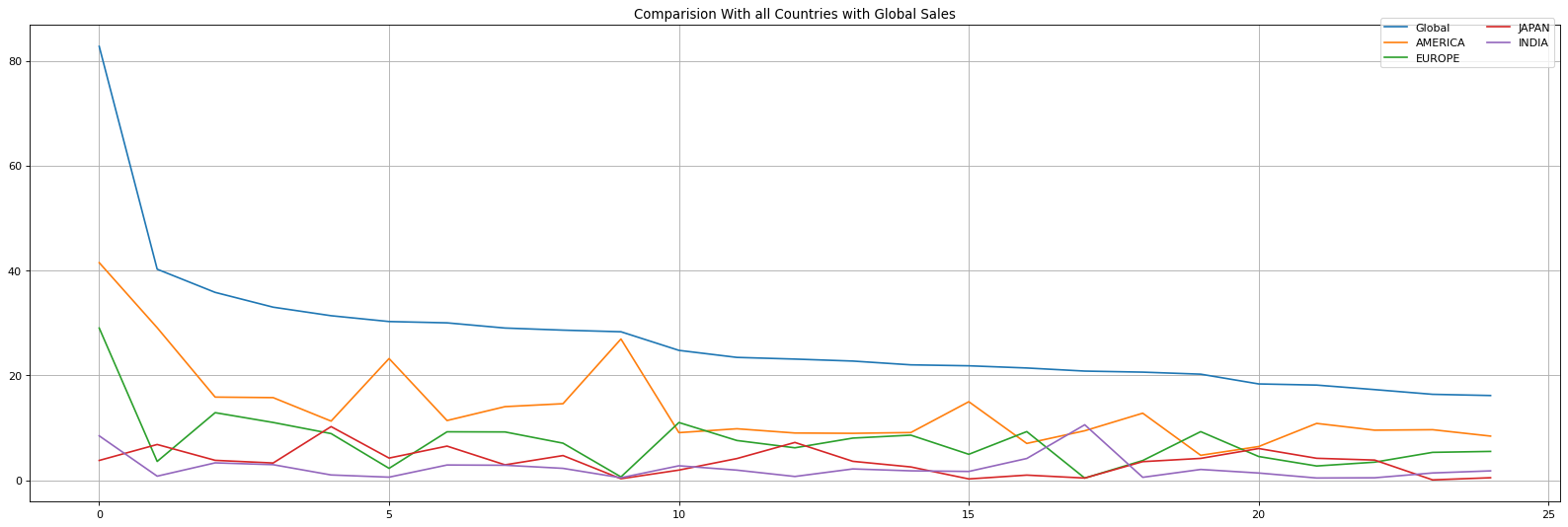In :
``````plt.figure(figsize=(25,8))
kwargs = dict(histtype='barstacked', alpha=0.3, bins=40)
plt.hist(total, **kwargs)
plt.hist(NA, **kwargs)
plt.hist(EUR, **kwargs)
plt.hist(JAP, **kwargs)
plt.hist(IND, **kwargs)
plt.xlabel('Global Sales')
plt.ylabel('Countries')
plt.title('Stepfield type of Comparision of Global with all Countries')``````
Out:
``Text(0.5, 1.0, 'Stepfield type of Comparision of Global with all Countries')``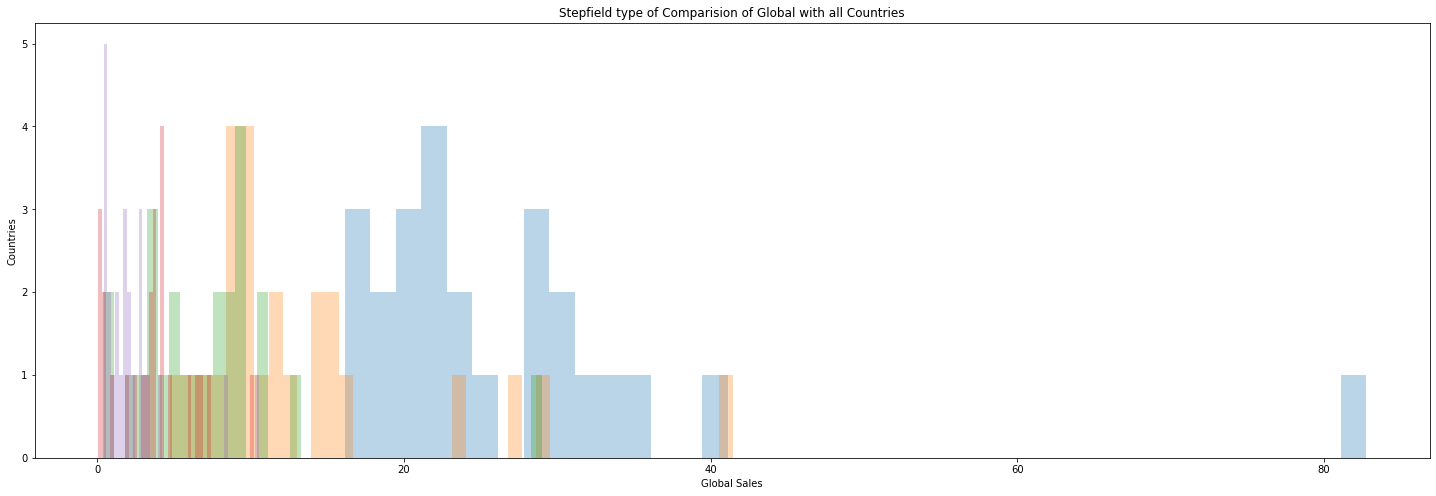In :
``````plt.figure(figsize=(10,7))
x = vg_plot['Year']
y = vg_plot['Global_Sales']
plt.title('Global sales occur (in Millions)')
plt.hist2d(x, y, bins=22, cmap='hot_r')
cb = plt.colorbar()
cb.set_label('counts in bin')``````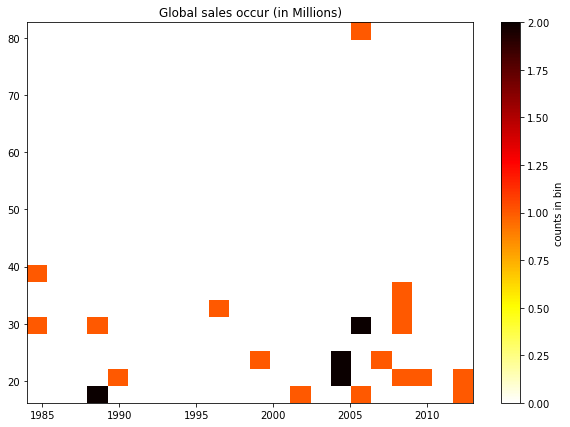## Exploring Seaborn Plots

The main idea of Seaborn is that it provides high-level commands to create a variety of plot types useful for statistical data exploration, and even some statistical model fitting. Let's take a look with our dataset 'videogame_df' and plot the types available in Seaborn.

### Maximum games sold using Countplot method

In :
``````plt.figure(figsize=(25,10))
sns.countplot('Year',data=videogame_df)
plt.title('Maximum Games sold on basis of Year')
plt.show()
``````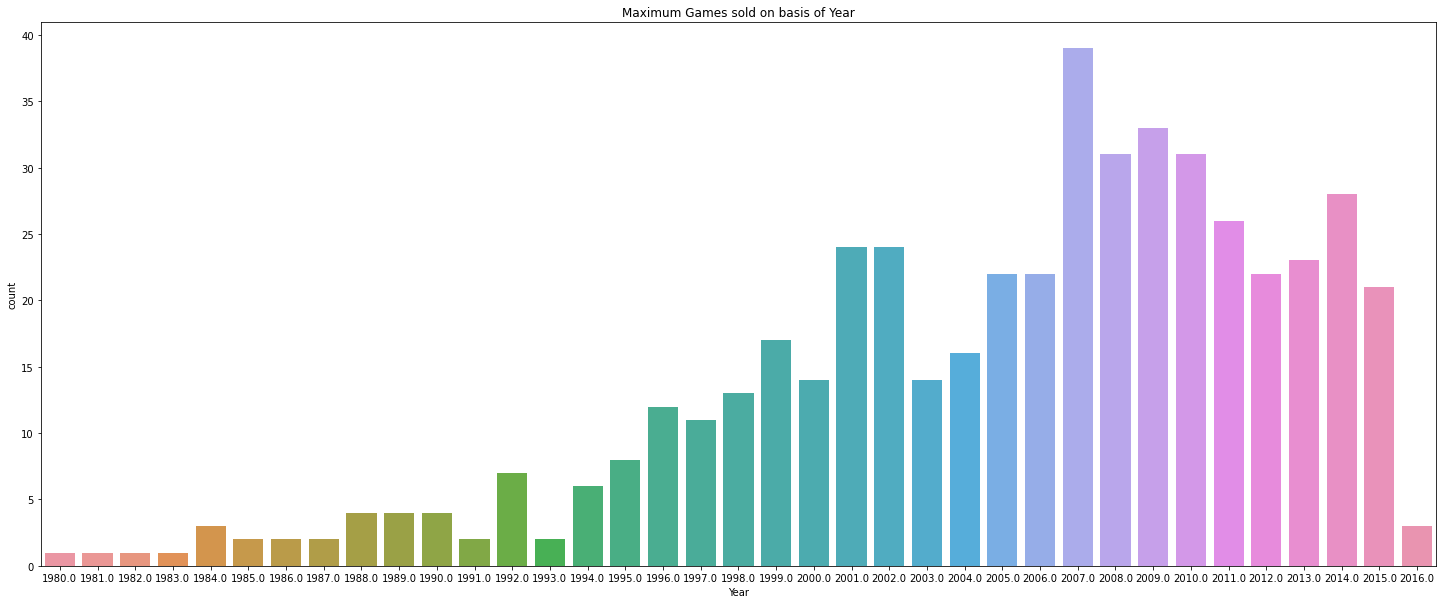### Top 10 Platforms, Genres, Publishers with Histogram plotting

In :
``````#top platforms (name of the platform,total number of games developed for that platform)

#top genres (name of the genre,total number of games developed in that genre)

#top game developers/publishers (name of the publisher,total number of games published by that publisher)

fig, (ax1,ax2) = plt.subplots(1,2,figsize=(25,8), facecolor='white')

##top platforms used for games
ax1.set_title('Top 10 Platforms',fontsize=16)

#top genres of Games accordingly
ax2.vlines(x=topGenres_index, ymin=0, ymax=topGenres_values, color='#AB0DD5', linewidth=30)
ax2.set_title('Top 10 Genres',fontsize=16)
plt.show()

fig, ax = plt.subplots(figsize=(25,8), facecolor='white')

#top publishers of the games
ax.vlines(x=topPublisher_index, ymin=0, ymax=topPublisher_values, linewidth=65, color='#969F79')
ax.set_title('Top 10 Publishers',fontsize=16)

``````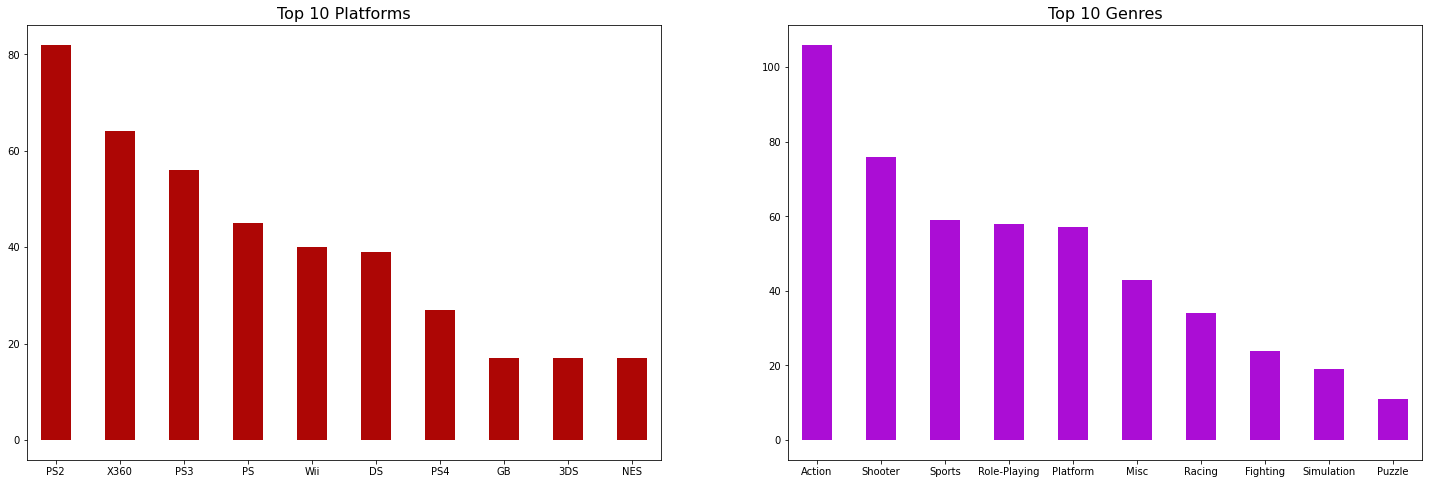Out:
``Text(0.5, 1.0, 'Top 10 Publishers')``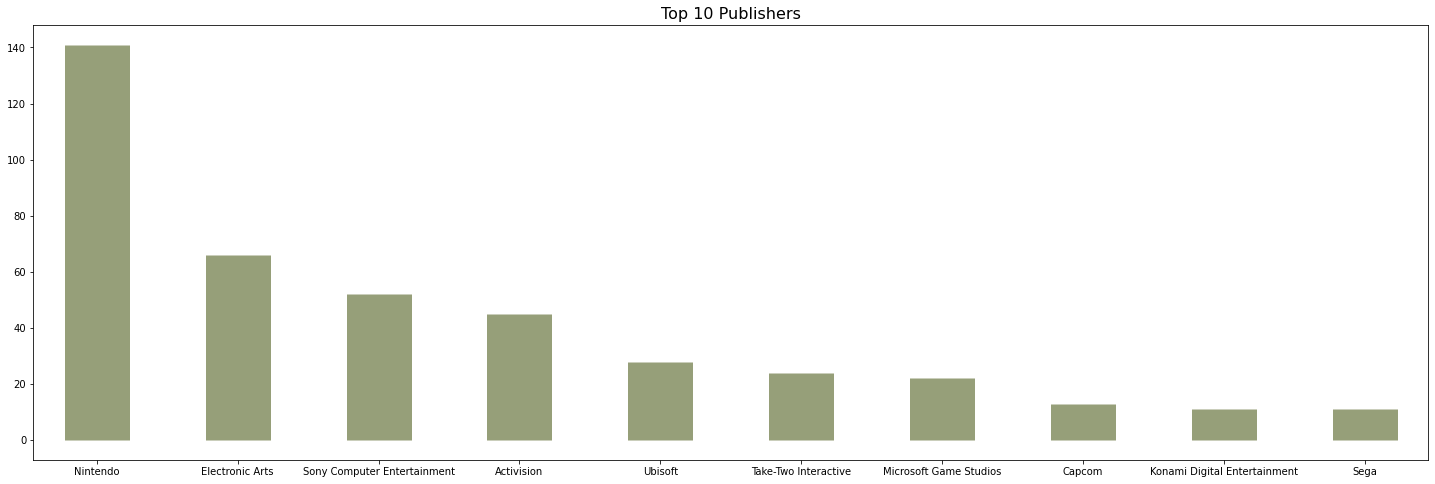Conclution for above Bar Graph are :

• DS and PS2 are the most popular platforms in comparison to others platform.
• Action is the most popular genre and the second most is the sports
• Electronic Arts has published 1300+ products

### Corellating the Games Sales among Countries and Global with Seaborn

Visualizing the multidimensional relationships among the samples is as easy as calling sns.pairplot:

In :
``````# Corellating among all Continents/Countries using Seaborn to perform pairplot and to plot the graph with matplotlib:

sns.pairplot(videogame_df.loc[0:,['NA_Sales','EUR_Sales','JAP_Sales','IND_Sales','Global_Sales']])
plt.show()
``````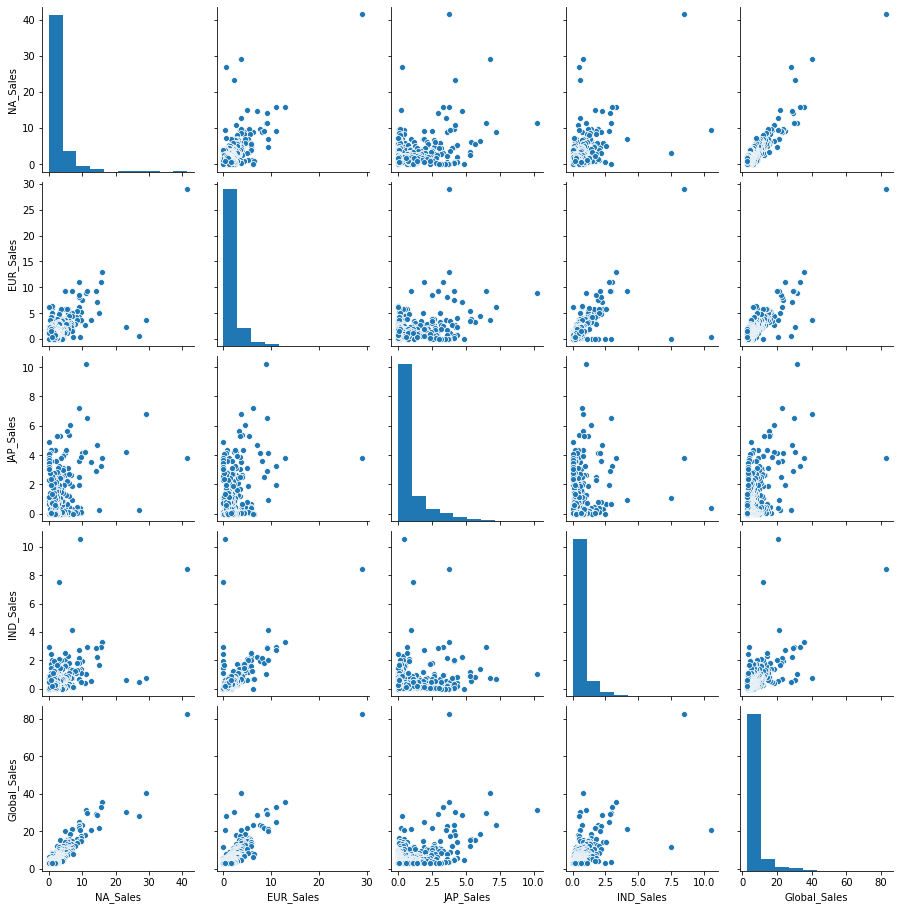Conclution Upon performing the correlation among various countries :

• North America is the major market as the Global sales are highly correlated with it.
• Europe is also an important region.
• One intresting thing is Japanies sales are not correlated with any region's sales,We can assume that JAPANIES people have different taste, when it's about games.

### TOP 15 GAMES IN INDIA USING BAR CHART (HORIZONTALLY)

In :
``````top15 = videogame_df[0:15]
top15
``````
Out:
In :
``````plt.figure(figsize = (18,8))
plt.barh(top15["Name"],top15["IND_Sales"], label = 'Top Games')
plt.title("Top 15 games sold in India",fontdict = {"fontsize":20})
plt.savefig("Top 15 games soldm in India.jpg",dpi = 300) #And to save it as an Jpeg image in the Directory
plt.legend()
plt.show()
``````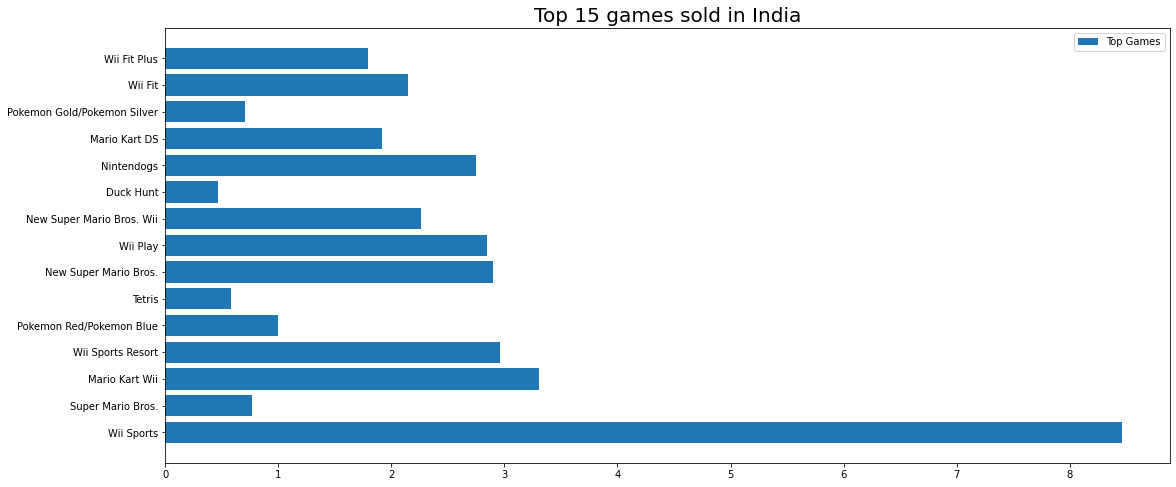### TOP 10 PUBLISHERS OF GAMES USING PIE CHART

In :
``````Publisher = list(videogame_df.Publisher.unique())
global_sale_of_every_Publisher = pd.Series(dtype = float)
for pub in Publisher :
data = videogame_df.loc[videogame_df.Publisher == pub]
global_sale = sum(data.Global_Sales)
global_sale_of_every_Publisher[pub] = global_sale
``````
In :
``top_10 = global_sale_of_every_Publisher[:10]``
In :
``````plt.figure(figsize = (10.5,9))
plt.pie(top_10,labels = top_10.index,autopct = "%.2f%%",textprops = {"fontsize":13},labeldistance = 1.05)
plt.legend(loc = 4,fontsize  = 12, bbox_to_anchor =(1.75, 0.82), ncol = 2)
plt.title("Top 10 Publisher of Games",fontdict = {"fontsize":25,"fontweight":100})
plt.savefig("Top 10 Publisher of Games",dpi = 200)
plt.show()
``````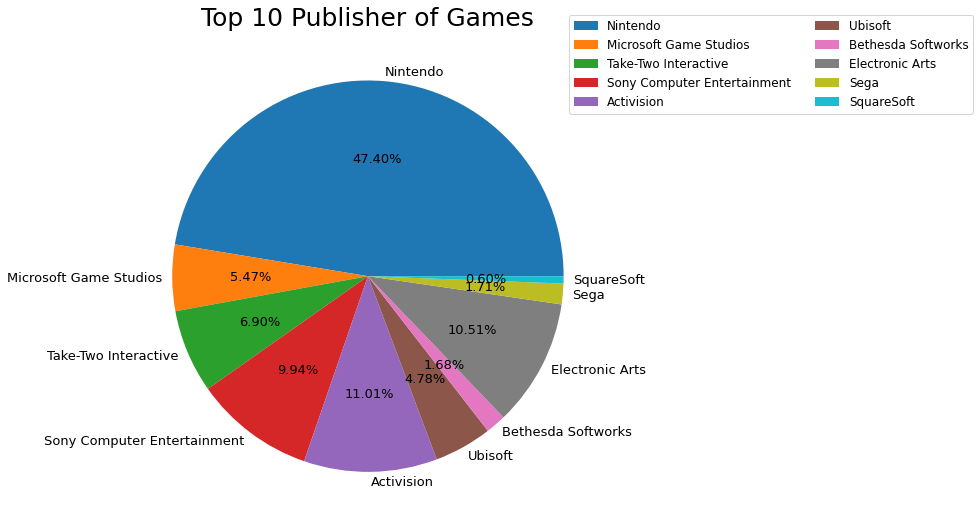### Percentage of Each Genre of Games

In :
``````Genre = videogame_df.Genre
Genre = Genre.value_counts()
``````
In :
``````plt.figure(figsize = (8,8))
labels = Genre.index
colors = ["#eeff00","#51ff00","#00ffdd","#ff9d00","#0033ff","#ff0800","#f700ff","#850012","#c7714a","#04615b","#ab8d5e","#00004a"]
plt.pie(Genre,labels = labels,colors = colors,autopct = "%.2f%%")
plt.title("Percentage of Top Genres of Games",fontdict = {"fontsize":17})
plt.savefig("Top Genres Chart",dpi = 200)
plt.show()
``````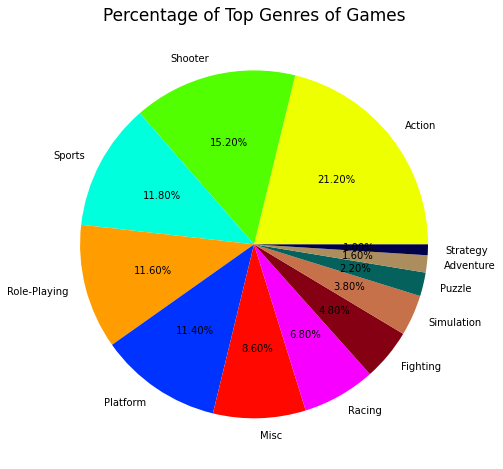### Best Selling Games in Countries

In :
``````#Pie Plot

# For North America
df1 = pd.DataFrame(videogame_df.groupby('Name')['NA_Sales'].sum())
df1.sort_values(by=['NA_Sales'], inplace=True)
df1 = df1.tail(5)
df1.plot.pie(y='NA_Sales', autopct='%1.1f%%', figsize=(6, 6))
plt.title("Best selling games in North America")

# For Europe Sales
df1 = pd.DataFrame(videogame_df.groupby('Name')['EUR_Sales'].sum())
df1.sort_values(by=['EUR_Sales'], inplace=True)
df1 = df1.tail(5)
df1.plot.pie(y='EUR_Sales', autopct='%1.1f%%', figsize=(6, 6))
plt.title("Best selling games in Europe")

# For India Sales
df1 = pd.DataFrame(videogame_df.groupby('Name')['IND_Sales'].sum())
df1.sort_values(by=['IND_Sales'], inplace=True)
df1 = df1.tail(5)
df1.plot.pie(y='IND_Sales', autopct='%1.1f%%', figsize=(6, 6))
plt.title("Best selling games in INDIA")

# For Japan Sales
df1 = pd.DataFrame(videogame_df.groupby('Name')['JAP_Sales'].sum())
df1.sort_values(by=['JAP_Sales'], inplace=True)
df1 = df1.tail(5)
df1.plot.pie(y='JAP_Sales', autopct='%1.1f%%', figsize=(6, 6))
plt.title("Best selling games in Japan")``````
Out:
``Text(0.5, 1.0, 'Best selling games in Japan')``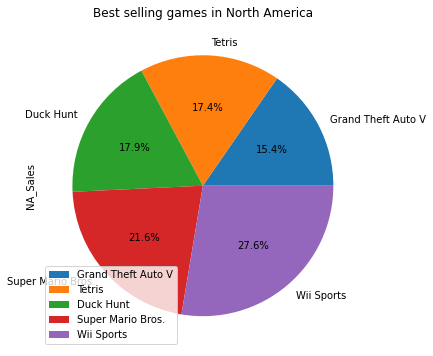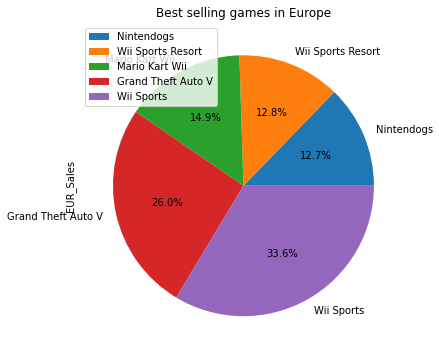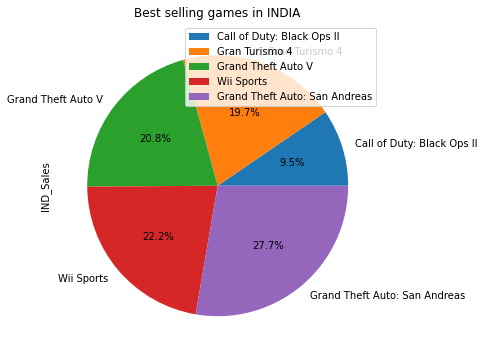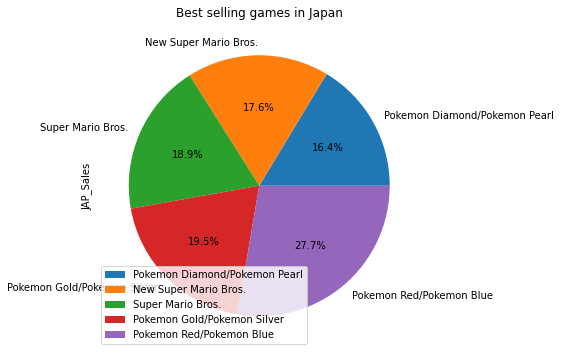### Video Game Sale Based on Genre (Global vs. INDIA)

In :
``````df_genre = videogame_df.groupby('Genre')
def genreBased(region):
xrange = np.arange(1,len(df_genre.sum())+1)
fig,ax= plt.subplots(ncols=2,figsize=(18,6))
df_to_plot = df_genre.sum().sort_values(by=region,ascending =False)[::-1]
df_to_plot[region].plot(kind='barh')
plt.title(region)
#labels
ax.set_ylabel(None)
ax.tick_params(axis='both', which='major', labelsize=13)
#spines
ax.spines['top'].set_visible(False)
ax.spines['right'].set_visible(False)
ax.grid(False)

#annotations
for x,y in zip(np.arange(len(df_genre.sum())+1),df_genre.sum().sort_values(by=region,ascending =False)[::-1][region]):
label = "{:}".format(y)
labelr = round(y,2)
plt.annotate(labelr, # this is the text
(y,x), # this is the point to label
textcoords="offset points",# how to position the text
xytext=(6,0), # distance from text to points (x,y)
ha='left',va="center")

#donut chart
theme = plt.get_cmap('Blues')
ax.set_prop_cycle("color", [theme(1. * i / len(df_to_plot))for i in range(len(df_to_plot))])
wedges, texts,_ = ax.pie(df_to_plot[region], wedgeprops=dict(width=0.45), startangle=-45,labels=df_to_plot.index,
autopct="%.1f%%",textprops={'fontsize': 13,})

plt.tight_layout()
``````
In :
``````genreBased('Global_Sales') #ABOVE
genreBased('IND_Sales') #BELOW``````